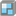• # via matrices

## Question related to missionGround for the House

Hi, my idea was to create matrix or list of lists. Then to find max and min value of cols and min and max value of rows. Than subtract min value of cols from max value of cols, e.g.: maxcol - mincol .The same action for rows, e.g maxrow - minrow.

To me it is not clear why when i iterate over matrix my loop jumps from col 0, to col 1, even it there are still "#". My code is here:

def house(plan):

```sharps = []
"Sharps is a list of pairs (coll,row) for each #"

matrix = [list(x) for x in plan.splitlines()]
# I don't know why, but matrix starts from empty list, hence need to remove empty list
# from the beginning
matrix = [col for col in matrix if len(col) != 0]

for row in range(len(matrix) - 1):
for col in range(len(matrix) - 1):
if matrix[row][col] == '#':
sharps.append((col, row))
print("col {} , row {}".format(col, row))
else:
col += 1

row += 1

print(matrix)
print(sharps)

print(house('''
0000000
##00##0
######0
##00##0
#0000#0
'''))
```8